# End Behavior in Math: Definition & Rules

An error occurred trying to load this video.

Try refreshing the page, or contact customer support.

Coming up next: Partial Derivative: Definition, Rules & Examples

### You're on a roll. Keep up the good work!

Replay
Your next lesson will play in 10 seconds
• 0:00 What Is End Behavior?
• 0:45 Positive Leading Term-Even
• 2:25 Negative Leading Term- Even
• 3:05 Positive Leading Term- Odd
• 3:30 Negative Leading Term- Odd
• 4:10 Lesson Summary

Want to watch this again later?

Timeline
Autoplay
Autoplay
Speed

#### Recommended Lessons and Courses for You

Lesson Transcript
Instructor: Ellen Manchester
All functions can be graphed. In this lesson, we will be looking at the end behavior of several basic functions. You will find that functions' leading terms indicate what kind of end behavior will be exhibited.

## What is End Behavior?

Every function can be drawn as a basic graph or 'picture'. Every graph has certain end behavior characteristics. The end behavior of a graph is defined as what is going on at the ends of each graph. In other words, in what direction are the ends of the graphs heading?

For instance, does the graph go up at the ends? Does the graph go down at the ends? The answer to these questions can be found within a function's leading term, or the term with the variable (x) with the highest exponent. As the function approaches positive or negative infinity, the leading term determines what the graph looks like as it moves towards infinity. This end behavior is consistent based on the leading term of the equation and the leading exponent.

## Positive Leading Term with an Even Exponent

In every function we have a leading term. To reiterate, the leading term is the term in the function with the highest exponent. If we have a function like, f(x) = x^2 + 2x + 3', the leading term is the x^2 since the exponent 2 is the highest exponent. If we have a function like, g(x) = x^2 + x^3 + x^4, the leading term is x^4, since the exponent 4 is the highest. We can rearrange the function so the exponents are in descending order: g(x) = x^4 + x^3 + x^2.

In both of these functions, we see the leading term has an even exponent. The leading terms are also both positive. These two qualities - a positive exponent and a positive term - determine the end behavior of the graph. Let's take a look at the graph for f(x) = x^2 + 2x + 3: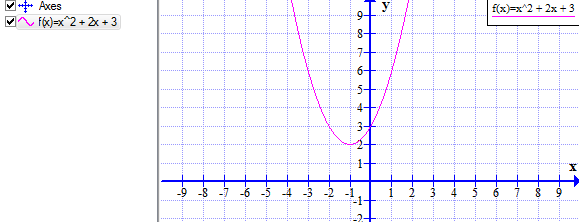What are the ends of this graph doing? Are the ends going up or down?

Now let's compare this graph with the graph of g(x) = x^4 + x^3 + x^2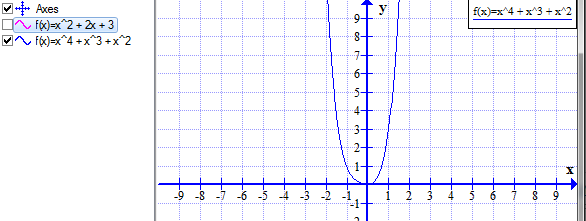What do you notice about the end behavior on this function? Did you notice the end behaviors of both graphs are doing the same thing? They are both going up at both ends. Let's look at one more function to see what the end behavior is doing.

Take a look at this graph of the function h(x) = x^8 - 3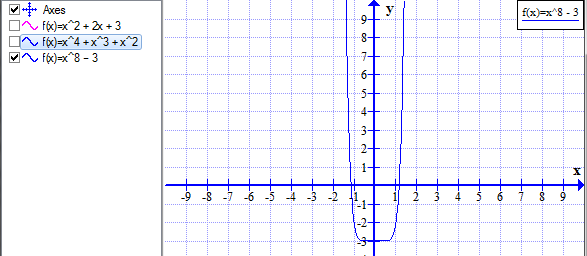Once more, when the leading exponent is an even number and the leading term is positive, the end behavior of the graph goes up at both ends.This is a set characteristic.

## Negative Leading Term with an Even Exponent

Now let's look at functions when the leading term is negative, but the exponent is still even. Before looking at the graphs, do you have a guess as to what you think the end behavior might be?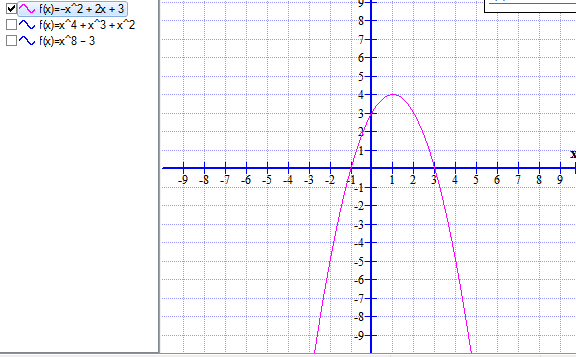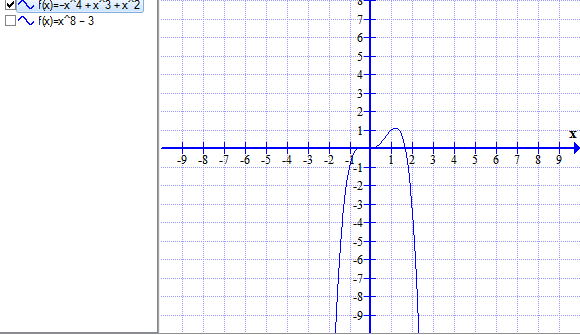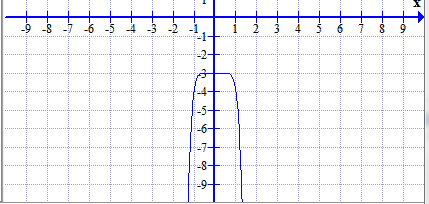The end behavior of the functions are all going down at both ends. This is because the leading coefficient is now negative. So, when you have a function where the leading term is negative with an even exponent, the ends of the function both go down.

You can remember it this way:

To unlock this lesson you must be a Study.com Member.

### Register to view this lesson

Are you a student or a teacher?

### Unlock Your Education

#### See for yourself why 30 million people use Study.com

##### Become a Study.com member and start learning now.
Back
What teachers are saying about Study.com

### Earning College Credit

Did you know… We have over 160 college courses that prepare you to earn credit by exam that is accepted by over 1,500 colleges and universities. You can test out of the first two years of college and save thousands off your degree. Anyone can earn credit-by-exam regardless of age or education level.